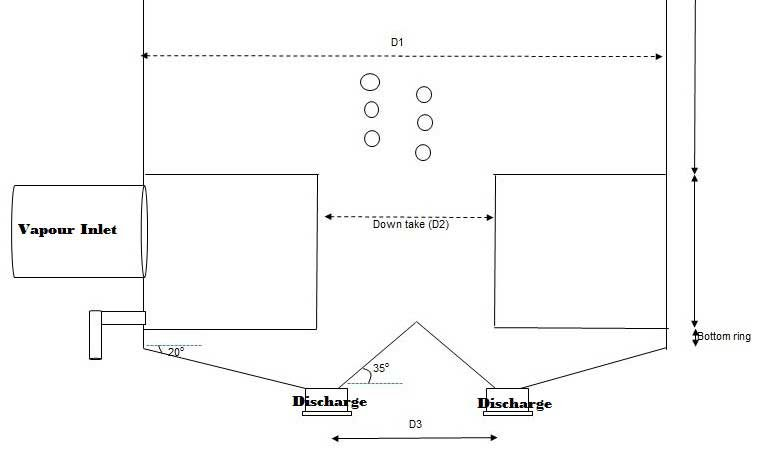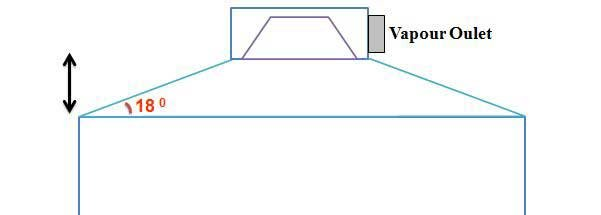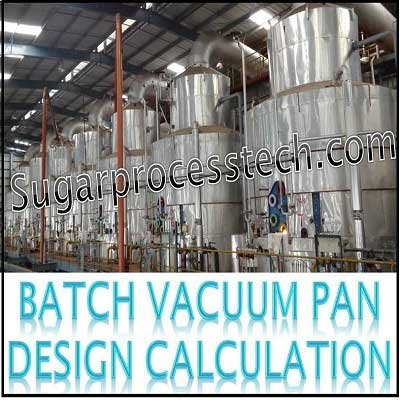# Batch Vacuum Pan Design Calculation | Sugarprocesstech | Sugar Technology

This article discussed about 80 MT capacity batch vacuum pan design calculation of Heating surface, Number of tubes, Dia of the downtake and tube plate, Graining volume, Dia of the vapour doom, Height of the top cone, Dia of vapour inlet and outlet pipe lines , Pipe line dia of noxious gases, Dia of condensate pipe line, Dia of Massecuite discharge, Calendria shell thickness and tube plate thicknesses

## Batch Vacuum Pan Calculation in sugar industry | Crystallization process

Capacity required for crystallization process  equipment like supply tanks, Capacity of Batch/continuous pans, condensers, molasses conditioners, spray pond, crystallizers capacity .. etc.

Please go throuh the below link for complete information regarding the above topics

Capacity Calculation of Pan Section

Basic design aspects of vacuum pan

### Design of 80 MT capacity Batch Pan

#### 1. Heating Surface :

Heating surface calculated on the basis of S/V ratio

For  batch pan with 3rd vapour  has a heating medium it will be 6.6 to 6.7 m2/m3

Formula = S/ V = 6.6

Srike volume = Weight/density of massecuite  = 80 MT / 1.42 MT / m3 =  56.33 m3 ≈ 57 m3 = 570 HL

Heating surface required = 57 x 6.6 = 376 m2

#### 2. Number of tubes

Generally, the specification of tubes for batch vacuum pan as follows

102 mm OD  / 16 g ( 1.625 mm) / 800 mm length

Formula : S = Π x D x L  x N

Here S = heating surface area of the pan in m2

D = Mean dia of tube in m  ( OD – thickness of tube )

D   = 102 – 1.625 = 100.375 mm

L = Effective Length of the tube in m ( Total length – 2 x Tube plate thickness – 2 x Tube expansion elevation and projection of the tube )

L = 800 -( 2 x 32) – (2 x 5) = 800 – 64 – 10 = 726 mm

N = Number of the tubes

So   N = 376 / ( 3.14 x 0.726 x 0.100375 ) = 1643 nos.

#### 3. Dia of the Downtake and tube plate:

Formula : Area of the tube plate =Here N = Number of the tubes

P = Pitch of the tube in m

Considered 20% extra area to arrange vapour distribution in calendria of batch vacuum pan

Here first we calculate dia required for down take

It is calculated on the basis of circulation ratio of the pan and it is generally maintained minimum 2.5Total cross section area of the tubes (A) in m2  = N x ( Π /4) x ID2

A = 1643 x 0.785 x ( 0.09875)2 = 12.58 m2

Area of the down take ( AD )in m2 = 12.58 / 2.5 = 5.032 m2

Dia of the down take == 2.53 m = 2500 mm

Pitch of the Tube

Legment of the tubes for vacuum pan = 16 mm

So Pitch of the tube = OD + legment + Tube tolerance + Hole tolerance

Pitch of the tube  P = 102 + 16 + 0.5 + 0.1 = 118.6 mm

Area of the tube plate A in m2= 1643 x 0.866 x (0.1186 )2 x 1. 2 + 5.032

A = 24.02 + 5.032 = 29.05 m2

Dia of the tube plate ( DTP) in m =DTP  = 6100 mm

Generally, dia of the downtake maintained 40% on tube plate dia for proper circulation of massecuite in pan.

Here 6100 x 40 % = 2440 mm ( As per above calculation it is 2500 mm. So it is OK )

#### 4. Graining volume the batch vacuum pan

Please go throuh the below link for complete information regarding this topicSl.no. Description Formula Values UOM Input Data 1 Capacity of pan 80 T 2 No. of tubes N 1643 nos. 3 Tube thickness t 1.6 mm 4 Tube Length H1 800 mm 5 Tube OD OD 102 mm 6 Dia of pan D1 6100 mm 7 Dia of the down take D2 2500 mm 8 Dia of bottom inverted cone D3 2200 mm 9 Height of the bottom ring H2 50 mm 10 Angle of bottom cone α 18 Deg 11 Angle of bottom inverted cone Φ 35 Deg Graining Volume Calculation 1 ID of the tube ID = OD – 2t 98.8 2 Volume of massecuite in tubes Q1 = 0.785 x ID x ID x H1 x N 10.07 M3 3 Volume of down take Q2 = 0.785 x D2 x D2 x H1 3.93 M3 4 Volume of the bottom ring Q3 = 0.785 x D1 x D1 x H2 1.46 M3 5 Height of the bottom cone h 1 = [(D1 – D3)/2 ] x TAN α 633.59 mm 6 A1 0.785 x (D1)2 29.21 M2 7 A2 0.785 x (D3)2 3.80 M2 8 Volume of the bottom cone Q4 = h/3 (A1+A2+√A1A2) 9.20 M3 9 Height of the bottom inverted cone h2 = [( D3)/2 ] x TAN Φ 770.23 mm 10 Volume of inverted cone Q5 = 1/3 x 0.785 x (D3)2 h2 0.98 M3 11 Graining Volume Q1+Q2+Q3+Q4 – Q5 23.68 M3 42.0 %

#### 5. Srike height:

Formula : Strick height ( Hs) in m = Volume of the massecuite above the tube plate in m3 / ( 0.785 x ID2 of the vapour space in m)

Here

Volume of the massecuite above the tube plate = Strike volume – graining volume

= 57 m3 – 23.68 m3 = 33.32 m3

ID of the vapour space = ID of the tube plate = 6100 mm  – ( 2 x thickness of the vapour shell)

= 6100 – (2 x 16)  = 6068 mm

Hs = 33.32 / [0.785 x (6.068)2] = 1.152 m = 1150 mm

#### 6. Dia of the vapour dome

Dia of the vapour doom in meters ( Dd )=Here

Volume of the vapour = Heating surface of pan x Evaporation rate x Specific volume of the vapour at 700 mm of Hg vacuum

Evaporation rate of the batch vacuum pans depends on type of the massecuites

Average evaporation rate in Batch Pans considered as follows

For ‘A ‘ Massecuite – 60 kg/m² /hr ,
For ‘B’ Massecuite – 55 kg/m² /hr
And for ‘ C’ Massecuite – 50 kg/m² /hr

So volume of the vapour = 376 x 60 x 11.04 =  249062 m3 /hr  ()

= 249062 / 3600 = 69.18 m3 /sec

Vapour velocity through the doom to be considered as 25 m/sec

Dd =  √ 1.27 x ( 69.18 / 25 )  = 1.874 m  ≈  1900 mm

#### 7. Height of the top cone part

Formula : Height of the top cone  HTC  =Here DTP  = ID of the tube plate in mm = 6100 – (2 x 16) = 6068 mm

Dd = Dia of the top doom = 1900  – (2 x 18) = 1864 mm
∝  = Angle of the cone = 18o ( Generally, it in the range of 18 to 20)

HTC = [ (6068 – 1864) /2 ] x Tan 18o

= 2102 x 0.325 = 683 mm

#### 8. Vapour inlet and outlet line dia

Vapour Inlet pipe dia =S.No Description Value UOM 1 Heating surface of the pan 376 m2 2 Evaporation rate 60 kgs/m2/hr 3 Pan Inlet Vapour Temperature 94 oC 4 Pan Outlet Vapour Temperature 52 oC 5 Pan Inlet Vapour Velocity 35 m/sec 6 Pan Outlet Vapour Velocity 55 m/sec Result 1 Specific volume of Pan inlet vapour pan boiling calculation2.0510 M3/kg 2 Specific volume of Pan Outlet vapour 10.98 M3/kg 3 Volume of the pan inlet vapour 12.853 M3/sec 4 Volume of the pan outlet vapour 68.81 M3/sec 5 Pan Inlet vapour Line Dia 0.684 mtrs Say 700 mm 6 Pan Outlet vapour Line Dia 1.262 mm Say 1300 mm

#### 9. Vapour space height (Free space above the massecuite level upto top cone )

Generally, Vapour space required above the massecuite level is minimum 1500 mm.

#### 10. Noxious gases connections

Generally,  For removal of non-condensable gases required 1 cm2 area for  10 m2   heating surface of vacuum pan

SO Cross section area of non-condensable gases pipe line in cm2 = Heating surface in m2 /10

= 376 /10  = 37.6 cm2

Dia of the each non condensable gases pipe line =Here considered 6 nos. of non condensable connections.

So Dia of the each non condensable gases pipe line  = √ 376 / (0.785 x 6 ) = 2.82 cm ≈ 32 mm

Non condensable gas connections = 32 mm x 6 nos.

#### 11. Dia of massecuite discharge

Dia of the massecuite discharge =Strike Volume = 56.33 m3

Time required for massecuite discharge = 10 to 15 min.

Velocity of massecuite = 0.15 m/sec

Dia of the massecuite discharge = √  56.33 / ( 0.785 x 12 x 60 x 0.15 ) = 0.815 m   ≈ 800 mm

#### 12. Dia of Condensate piping

Generally, for 80 MT pan condensate withdrawal points required minimum 2 nos. It is better to go with 3 nos. of  connections.

Dia of the each condensate line =Volume of the condensate =  [Heating surface X Evap. Rate ] / [ Density of water x 3600].

= 376 x 60 / ( 1 x 3600 )

= 22.560 / 3600 = 0.00627 m3 /sec

Dia of the each condensate line = √  0.00627 / ( 0.785 x 1 x  3  ) = 0.052  ≈ 80 mm

#### 13. Calendria shell thickness

Formula : Calendria shell thickness ( ts )  in mm =Here

P = Hydraulic test pressure  in kg/cm2    = 3 kg/cm2

Di = ID of the Calendria in mm  = 6100 – ( 2 x 16) = 6068 mm ( Here 16 mm considered for shell thickness for calculation purpose)

F = Allowable stress in kg/cm = For Mild steel it sis considered as 1400 kg/cm2

J = Welding Joint efficiency in mm = 0.75 mm

C= corrosion allowance in mm  = 3.0 mm

t s ={ 3.0 x 6068 / [ ( 2 x 1400 x 0.75 ) – 3 ] } + 3  = 12 mm

But according to standard specifications  calendria and body shell plate thickness for 80 MT pan is 18 mm and for bottom saucer is 25 mm

#### 14. Tube Plate thickness

Tube plate thickness  ( tp) in mm =Here  F =K =Here

C . A = corrosion allowance in mm  = 1.5 mm

f  = Allowable stress in kg/cm= 1400kg/cm2

P = Design pressure in kg/cm2 = 2.72  kg/cm2

Es = Modulus factor for MS sheet in kg/cm= 2.1 x 106 Kg/cm2

Et = Modulus factor for SS sheet in kg/cm= 1.9 x 106 Kg/cm2

G = ID of the shell in mm  = 6068 mm

ts = Thickness of the shell in mm  = 18 mm

tt = Thickness of tube in mm = 1.625 mm

do= OD of the tube in mm = 102 mm

Do = OD of the calendria sheet in mm  = 6100 mm

Nt = Number of tubes = 1643 nos.

K =K = 0.4515

F = √  0.4515 / [ 2 + 3(0.4515)] = 0.3669

tp = 0.3669 x 6068 x √ (0.25 x 2.75 /1400) + 1.5 = 50.56 mm

According to standard specification, 36 mm thickness is sufficient for 80 MT  tube plate.Related Articles:

Hydrostatic pressure definitions | Hydrostatic Head in Vacuum pans

Vertical Crystallizer Concepts in Sugar Plant | Mono Vertical Crystallizer

Cooling and reheating Process of low grade (B & C) massecuites

Sugar Seed Slurry Requirement Calculation for B and C massecuite

Types of graining techniques in sugar industry pan boiling

Three and half massecuite boiling material balance calculation

Sugar Factory Material Balance Calculation for three massecuite Boiling

Specific speed of a centrifugal pump | Formula for Pump Power Calculation

## 18 thoughts on “Batch Vacuum Pan Design Calculation | Sugarprocesstech | Sugar Technology”#### Patil shivaji Shankar

(May 27, 2019 - 4:06 pm)

Vry useful information#### siva alluri

(May 30, 2019 - 5:07 pm)

Thank you#### Uday Pratap Singh

(June 14, 2019 - 6:19 am)

Dear Sir,#### siva alluri

(June 14, 2019 - 4:22 pm)

OK we will provide soon#### Rambhau Dhawale

(May 28, 2020 - 9:05 am)

Must and nice discription is ok#### siva alluri

(June 21, 2020 - 9:57 am)

Thank you#### shashikant

(July 19, 2020 - 9:56 am)

thanks for such a valuable information ….thanks sir .#### siva alluri

(July 23, 2020 - 3:40 am)

welcome#### shashikant

(July 20, 2020 - 1:12 pm)

in tube plate thickness in f= underroot K/f+3K …….there is value taken f= 2 ….sir please help why this value#### siva alluri

(July 23, 2020 - 4:28 am)

F = shell side pressure. This factor verifying with heat exchanger#### paritoshVashishtha

(July 21, 2020 - 12:14 pm)

It’s really great, sir how to calculate steam consumption for DEVC plus Quintuple with five comparment cigar calculation#### Skgupta

(October 9, 2020 - 12:07 am)

Kindly provide calculations of umbrella below save all(November 1, 2020 - 2:43 am)

Thanks for such a valuable information ….thanks sir#### siva alluri

(November 1, 2020 - 1:23 pm)

Welcome(August 17, 2021 - 4:22 am)

Please provide S/V ratio calculation for batch and continuous pan#### siva alluri

(September 2, 2021 - 8:30 am)

For batch pan the S/V ratio will be in the range of 6.5 to 6.8 m2/m3

For continuous pan the S/V ratio will be in the range of 9.5 to 10.5 m2/m3#### Pandurang chavan

(July 14, 2023 - 10:19 am)

Ok